# Resistor Color Code Calculator

Resistor Color Code Calculator

HOW TO CALCULATE RESISTOR VALUE?

4 Band Resistor

1th Band First significant digit

2nd Band Second significant digit

3rd Band Multiplier

4th Band Tolerance

3rd Band and Temperature Coefficient leave empty!

5 Band Resistor

1th Band First significant digit

2nd Band Second significant digit

3rd Band Third significant digit

4th Band Multiplier

5th Band Tolerance

Temperature Coefficient leave empty!

6 Band Resistor

1th Band First significant digit

2nd Band Second significant digit

3rd Band Third significant digit

4th Band Multiplier

5th Band Tolerance

6th Band Temperature Coefficient (Tempco)

• For resistor calculator, enter the colors on the resistor above.
• The last two bands that are on 4 and 5 colored resistors are MULTIPLIER and TOLERANCE
• If the resistor is 4 colored, leave the 3rd band and temperature coefficient empty.
• If the resistor is 5 colored, leave only the temperature coefficient empty.

## Resistor Color Chart

 COLORS Coefficients Multiplier Tolerance Temperature Coefficient (Tempco) 1. band 2. band 3. band Black 0 0 1 Brown 1 1 1 10 ± %1 100 ppm Red 2 2 2 100 ± %2 50 ppm Orange 3 3 3 1k 15 ppm Yellow 4 4 4 10k 25 ppm Green 5 5 5 100k ± %0.5 Blue 6 6 6 1M ± %0.25 Violet 7 7 7 10M ± %0.10 Grey 8 8 8 ± %0.05 White 9 9 9 Gold 0.1 ± %5 Silver 0.01 ± %10 Colorless ± %20

### 4 BAND RESISTOR SAMPLES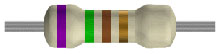4 Band Resistor Calculate

Coefficient = Violet (7), Green (5)Multiplier = Brown (1)Tolerance = Gold (%5)

Resistor Value = 75×101 = 750 Ohms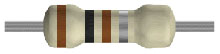4 Band Resistor Calculate

Coefficient = Brown (1), Black (0)Multiplier = Brown (1)Tolerance = Silver (%10)

Resistor Value = 10×101 = 100 Ohms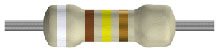4 Band Resistor Calculate

Coefficient = White (9), Brown (1)Multiplier = Yellow (4)   Tolerance = Gold (%5)

Resistor Value = 91×104 = 910 kOhms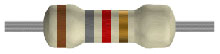4 Band Resistor Calculate

Coefficient = Brown (1), Grey (8)Multiplier = Red (2)Tolerance = Gold (%5)

Resistor Value = 18×102 = 1.8 kOhms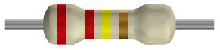4 Band Resistor Calculate

Coefficient = Red (2), Red (2)Multiplier = Yellow (4)Tolerance = Gold (%5)

Resistor Value = 22×104 = 220 kOhms

### 5 BAND RESISTOR SAMPLES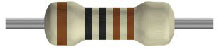5 Band Resistor Calculate

Coefficient = Brown (1), Black (0), Black (0)Multiplier = Brown (1)Tolerance = Brown (%1)

Resistor Value = 100×101 = 1 kOhms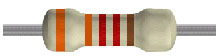5 Band Resistor Calculate

Coefficient = Orange (3), Orange (3), Red (2)Multiplier = Red (2)Tolerance = Brown (%1)

Resistor Value = 332×102 = 33.2 kOhms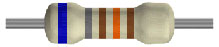5 Band Resistor Calculate

Coefficient = Blue (6), Grey (8), Brown (1)Multiplier = Orange (3)Tolerance = Brown (%1)

Resistor Value = 681×103 = 681 kOhms

### 6 BAND RESISTOR SAMPLE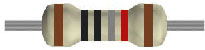6 Band Resistor Calculate

Coefficient = Brown (1), Black (0), Black (0)Multiplier = Silver (0.01)Tolerance = Red (%2)

ppm = Brown (100 ppm)

Resistor Value = 100×10-2 = 1 kOhms